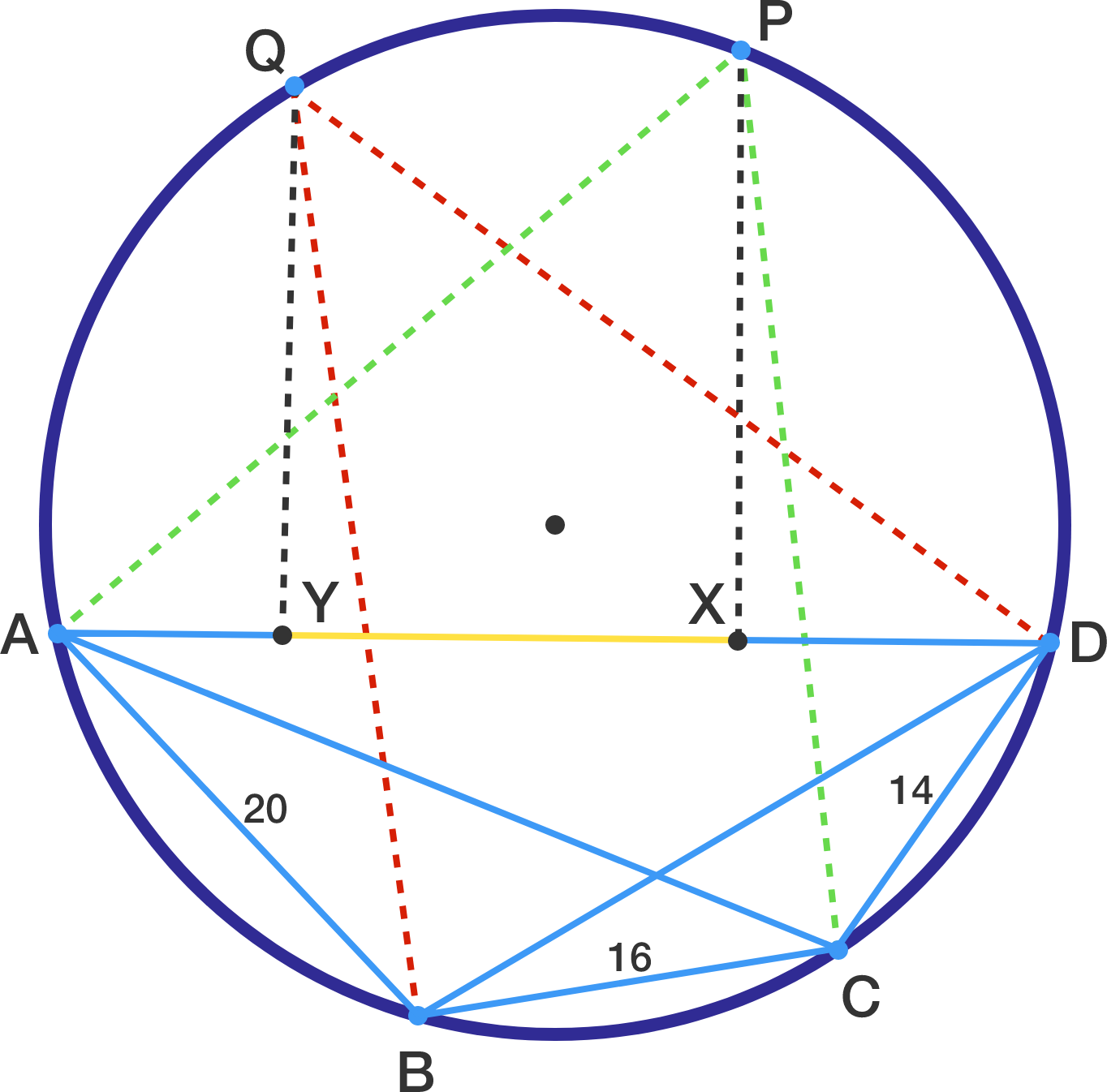# Perps in a Cyclic Quad

Geometry Level 3Let $ABCD$ be a cyclic quadrilateral with $\angle ABD = \angle ACD >90^{\circ}$.

Let $P$ and $Q$ be the points on major arc $AD$ satisfying $PA=PC$ and $QB=QD$.

Furthermore, let $X$ and $Y$ be the feet of the perpendiculars from $P$ and $Q$ to line $AD$.

If $AB=20$, $CD=14$, and $BC=16$, then find the length of $XY$.

×

Problem Loading...

Note Loading...

Set Loading...